# Team:Stockholm/model-simulation

<!doctype html>iGEM Stockholm Model Simulation

# Model Simulation

## P2 genetic switch

We first simulated C protein concentration dynamics in P2 genetic switch system over time using the final derived deterministic model:

$$\frac{dx}{dt\prime}=\frac{1+\tau u x^2}{1+ux^2+\sigma u^2x^4}-\gamma x=f(x)$$

We selected a time step of dt=0.01, as well as arbitrary parameters σ=2, u=1, γ=3, τ=2 and initial concentration of x=0.8. The following time series graph shows the stabilization of the protein concentration to a steady state.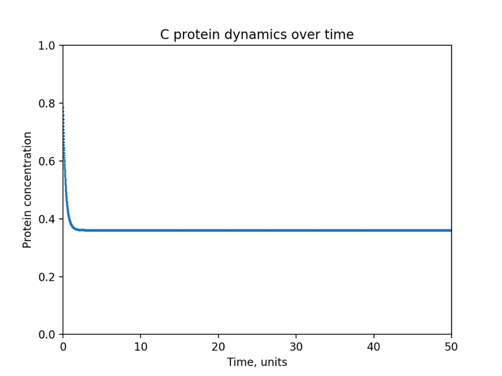Figure 1. C protein concentration over time in phage P2 switch system, deterministic model. σ=2, u=1, γ=3, τ=2, x0=0.8.

In order to determine the steady state concentration, we examine the f(x) versus x graph, from which we can tell the point where the graph crosses horizontal axis, corresponding to dx/dt=0, i.e. the steady state concentration. We determine this concentration being ~ 0.371.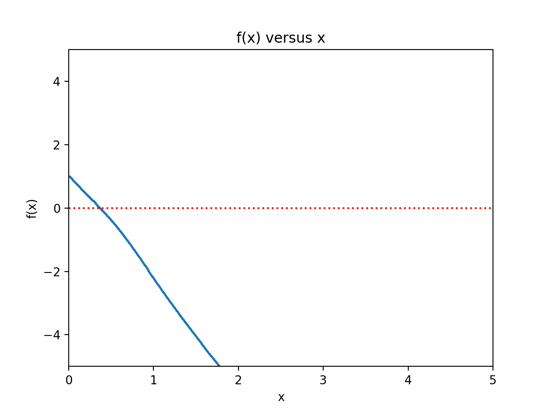Figure 2. f(x) versus x graph of deterministic P2 switch model. σ=2, u=1, γ=3, τ=2, x0=0.8.

Simulating the model adapted to a stochastic setting results in a similar graph, which, however, includes more fluctuations due to random noise, introduced into the model.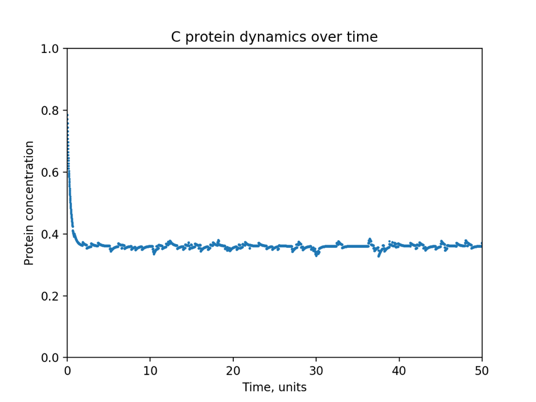Figure 3. C protein concentration over time in phage P2 switch system, stochastic model. σ=2, u=1, γ=3, τ=2, x0=0.8.

To our knowledge, this is the first attempt to derive and simulate a model for P2 bacteriophage genetic switch.

### Model Plasmid

We first simulated C and Cox protein concentration dynamics in Model Plasmid system over time using the final derived deterministic model:

$$\frac{dx}{dt}=\frac{K_{t1}K_1[arabinose]}{1+arabinose}-αx$$

$$\frac{dm}{dt}=\frac{arabinose}{1+arabinose}-βm$$

We selected a time step of dt=0.01, as well as arbitrary parameters σ=2, u=1, γ=3, τ=2 and initial concentration of x=0.5. The following time series graph shows the stabilization of all C and Cox protein concentrations to a steady state.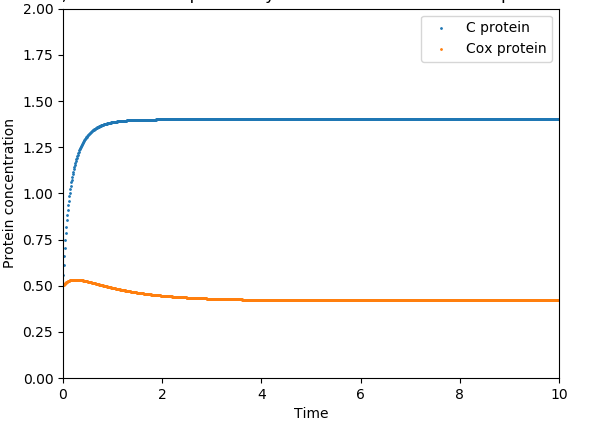Figure 4. C and Cox protein dynamics over time in the Model Plasmid.

It depicts that C protein increases exponentially up to a certain concentration (x=1.75) and stabilizes after a certain time point, in this case t= 1 unit. Cox protein seem to be almost stabilised throughout by t=2 .

In order to determine the steady state concentration, we examine the f(x) versus x [dx/dt v/s protein concentration] graph, from which we can tell the point where the graph crosses horizontal axis, corresponding to dx/dt=0, i.e. the steady state concentration.

We determine this concentration for C protein= 1.1 and Cox protein = 0.9

Simulating the model adapted to a stochastic setting results in a similar graph, which, however, includes more fluctuations due to random noise, introduced into the model.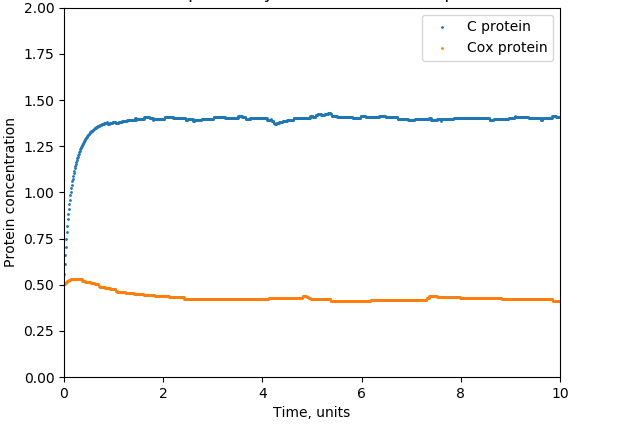Figure 6. C and Cox protein dynamics in Model Plasmid over time, stochastic model.

### Switch Plasmid

We first simulated C, Cox and TetR protein concentration dynamics in Switch Plasmid system over time using the final derived deterministic model:

$$\frac{dx}{dt}=\frac{k_{t1}+k_{t3}K_1K_Dr^2}{1+K_3[arabinose]+K_1K_Dr^2+K_D^2K_1K_2r^4}-αx$$

$$\frac{dm}{dt}=\frac{k_{t2}K_3[arabinose]}{1+K_3[arabinose]+K_1K_Dr^2+K_D^2K_1K_2r^4}-βx$$

$$\frac{dr}{dt}=\frac{k_{t2}K_3[arabinose]}{1+K_3[arabinose]+K_1K_Dr^2+K_D^2K_1K_2r^4}-γx$$

We selected a time step of dt=0.01, as well as arbitrary parameters σ=2, u=1, γ=3, τ=2 and initial concentration of x=0.5. The following time series graph shows the stabilization of all the three protein concentrations to a steady state.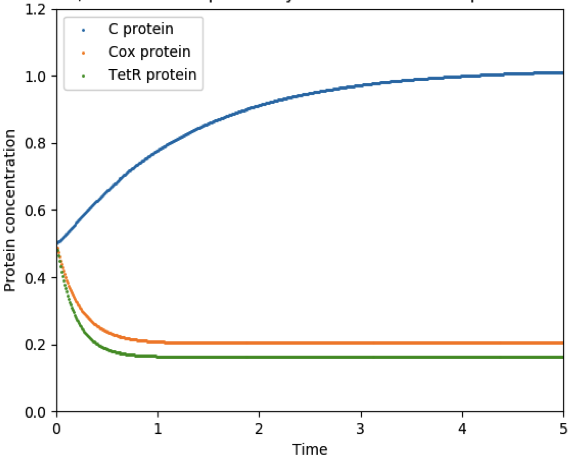Figure 7. C, Cox, TetR protein dynamics over time in the Switch Plasmid.

It depicts that C protein increases exponentially up to a certain concentration (x=0.8) and stabilizes after a certain time point, in this case t= 2 units. Cox and TetR protein seem to decrease in concentration up to a certain time point (t=0.5) and stabilizes at t=1.

In order to determine the steady state concentration, we examine the f(x) versus x [dx/dt v/s protein concentration] graph, from which we can tell the point where the graph crosses horizontal axis, corresponding to dx/dt=0, i.e. the steady state concentration.